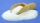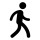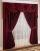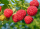# Fraction calculator

The calculator performs basic and advanced operations with fractions, expressions with fractions combined with integers, decimals, and mixed numbers. It also shows detailed step-by-step information about the fraction calculation procedure. Solve problems with two, three, or more fractions and numbers in one expression.

## Result:

### 61/5 - 41/4 = 39/20 = 1 19/20 = 1.95

Spelled result in words is thirty-nine twentieths (or one and nineteen twentieths).

### How do you solve fractions step by step?

1. Conversion a mixed number 6 1/5 to a improper fraction: 6 1/5 = 6 1/5 = 6 · 5 + 1/5 = 30 + 1/5 = 31/5

To find new numerator:
a) Multiply the whole number 6 by the denominator 5. Whole number 6 equally 6 * 5/5 = 30/5
b) Add the answer from previous step 30 to the numerator 1. New numerator is 30 + 1 = 31
c) Write a previous answer (new numerator 31) over the denominator 5.

Six and one fifth is thirty-one fifths
2. Conversion a mixed number 4 1/4 to a improper fraction: 4 1/4 = 4 1/4 = 4 · 4 + 1/4 = 16 + 1/4 = 17/4

To find new numerator:
a) Multiply the whole number 4 by the denominator 4. Whole number 4 equally 4 * 4/4 = 16/4
b) Add the answer from previous step 16 to the numerator 1. New numerator is 16 + 1 = 17
c) Write a previous answer (new numerator 17) over the denominator 4.

Four and one quarter is seventeen quarters
3. Subtract: 31/5 - 17/4 = 31 · 4/5 · 4 - 17 · 5/4 · 5 = 124/20 - 85/20 = 124 - 85/20 = 39/20
For adding, subtracting, and comparing fractions, it is suitable to adjust both fractions to a common (equal, identical) denominator. The common denominator you can calculate as the least common multiple of both denominators - LCM(5, 4) = 20. In practice, it is enough to find the common denominator (not necessarily the lowest) by multiplying the denominators: 5 × 4 = 20. In the next intermediate step, the fraction result cannot be further simplified by canceling.
In words - thirty-one fifths minus seventeen quarters = thirty-nine twentieths.

#### Rules for expressions with fractions:

Fractions - use the slash “/” between the numerator and denominator, i.e., for five-hundredths, enter 5/100. If you are using mixed numbers, be sure to leave a single space between the whole and fraction part.
The slash separates the numerator (number above a fraction line) and denominator (number below).

Mixed numerals (mixed fractions or mixed numbers) write as non-zero integer separated by one space and fraction i.e., 1 2/3 (having the same sign). An example of a negative mixed fraction: -5 1/2.
Because slash is both signs for fraction line and division, we recommended use colon (:) as the operator of division fractions i.e., 1/2 : 3.

Decimals (decimal numbers) enter with a decimal point . and they are automatically converted to fractions - i.e. 1.45.

The colon : and slash / is the symbol of division. Can be used to divide mixed numbers 1 2/3 : 4 3/8 or can be used for write complex fractions i.e. 1/2 : 1/3.
An asterisk * or × is the symbol for multiplication.
Plus + is addition, minus sign - is subtraction and ()[] is mathematical parentheses.
The exponentiation/power symbol is ^ - for example: (7/8-4/5)^2 = (7/8-4/5)2

#### Examples:

adding fractions: 2/4 + 3/4
subtracting fractions: 2/3 - 1/2
multiplying fractions: 7/8 * 3/9
dividing Fractions: 1/2 : 3/4
exponentiation of fraction: 3/5^3
fractional exponents: 16 ^ 1/2
adding fractions and mixed numbers: 8/5 + 6 2/7
dividing integer and fraction: 5 ÷ 1/2
complex fractions: 5/8 : 2 2/3
decimal to fraction: 0.625
Fraction to Decimal: 1/4
Fraction to Percent: 1/8 %
comparing fractions: 1/4 2/3
multiplying a fraction by a whole number: 6 * 3/4
square root of a fraction: sqrt(1/16)
reducing or simplifying the fraction (simplification) - dividing the numerator and denominator of a fraction by the same non-zero number - equivalent fraction: 4/22
expression with brackets: 1/3 * (1/2 - 3 3/8)
compound fraction: 3/4 of 5/7
fractions multiple: 2/3 of 3/5
divide to find the quotient: 3/5 ÷ 2/3

The calculator follows well-known rules for order of operations. The most common mnemonics for remembering this order of operations are:
PEMDAS - Parentheses, Exponents, Multiplication, Division, Addition, Subtraction.
BEDMAS - Brackets, Exponents, Division, Multiplication, Addition, Subtraction
BODMAS - Brackets, Of or Order, Division, Multiplication, Addition, Subtraction.
GEMDAS - Grouping Symbols - brackets (){}, Exponents, Multiplication, Division, Addition, Subtraction.
Be careful, always do multiplication and division before addition and subtraction. Some operators (+ and -) and (* and /) has the same priority and then must evaluate from left to right.

## Fractions in word problems:

• Fractions mul add sumTo three-eighths of one third, we add five quarters of one half and multiply the sum by four. How much will we get?
• Sugar 8Heather has 2 cups of powdered sugar. She sprinkles 3/5 of the sugar onto a plate of brownies and sprinkles the rest into a plate of lemon cookies. How much sugar does Heather sprinkle on the brownies? How much sugar does Heather sprinkle on the lemon coo
• Pupils 7There are 40 pupils in a certain class. 3/5 of the class are boys. How many are girls?
• Visit to grandmotherRobert's family traveled 5/8 of the distance to his grandmother’s house on Saturday. They traveled 1/3 of the remaining distance on Sunday. What fraction of the total distance to his grandmother’s house was traveled on Sunday?
• Interior designerTo make draperies an interior designer needs 11 1/4 yards of material for the den and 8 1/2 yards for the living room. If material comes only in 20 yard bolts, how much will be left over after completing both sets of draperies?
• Bitoo and ReenaBitoo ate 3/5 part of an apple and the remaining part was eaten by his sister Reena. How much part of an apple did Renna eat? Who had the larger share? By how much?
• SchoolThere are 150 pupils in grade 5 . 2/3 of them are female. By what fractions are the males?
• Mixed numbersRewrite mixed numbers, so the fractions have the same denominator: 5 1/5 - 2 2/3
• EricaErica bought 3 1/2 yards of fabric. If she uses 2/3 of the fabric, how much will she have left?
• KingKing had four sons. First inherit 1/2, second 1/4, third 1/5 of property. What part of the property was left to the last of the brothers?
• Find the 24Find the difference between 2/7 and 1/21
• CarrieCarrie picked 2/5 of the raspberries from the garden, and Robin picked some too.  When they were finished, 1/3 of the raspberries still needed to be picked.  What fraction of the raspberries did Robin pick? Use pictures, numbers or words and write your fi
• Ali bought 2Ali bought 5/6 litre of milk. He drank 1/2 litre and his brother drank 1/6 litre. How much litre of milk left?